# Greatest common divisor (GCD) + reason - math problems

#### Number of problems found: 22

• Lcm = 22 + gcdThe least common multiple of two numbers is 22 more than their greatest common divisor. Find these numbers.
• Athletics teamAll athletes in the athletics team from Ostrava can start in four, five, six and seven stages, and no one will be present. How many average athletes are in one athletic group if there are a total of twelve groups in the section? Consider the smallest numb
• We bought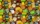We bought 140 fruit, 196 chocolate and 84 caramel lollipops. How many of the same packages can we prepare from them?
• Coloured numbersMussel wrote four different natural numbers with coloured markers: red, blue, green and yellow. When the red number divides by blue, it gets the green number as an incomplete proportion, and yellow represents the remainder after this division. When it div
• Three linesAt 6 am, three bus lines are departing from the station. The first line has an interval of 24 minutes. The second line has an interval of 15 minutes. The third line runs at regular intervals of more than 1 minute. The third line runs at the same time as t
• The ticketsThe tickets to the show cost some integer number greater than 1. Also, the sum of the price of the children's and adult tickets, as well as their product, was the power of the prime number. Find all possible ticket prices.
• Long bridge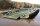Roman walked on the bridge. When he heard the whistle, he turned and saw running Kamil at the beginning of the bridge. If he went to him, they would meet in the middle of the bridge. Roman, however, rushed and so did not want to waste time returning 150m.
• Reminder and quotientThere are given the number C = 281, D = 201. Find the highest natural number S so that the C:S, D:S are with the remainder of 1,
• Four poplarsFour poplars are growing along the way. The distances between them are 35 m, 14 m, and 91 m. At least how many poplars need to be dropped to create the same spacing between the trees? How many meters will it be?
• Bricks pyramidHow many 50cm x 32cm x 30cm brick needed to built a 272m x 272m x 278m pyramid?
• Trees in alleyThere are four trees in the alley between which the distances are 35m, 15m and 95m. Trees must be laid in the spaces so that the distance is the same and the maximum. How many trees will they put in and what will be the distance between them?
• Hectares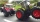The tractor plows the first day of 4.5 ha, the second day 6.3 ha, and the third day of 5.4 ha. It worked whole hours a day, and its hourly performance did not change and was the highest of the possible. How many hectares did it plow in one hour (what is i
• MO C–I–1 2018An unknown number is divisible by just four numbers from the set {6, 15, 20, 21, 70}. Determine which ones.
• Three friendsThree friends had balls in ratio 2: 7: 4 at the start of the game. Could they have the same number of balls at the end of the game? Write 0, if not, or write the minimum number of balls they had together.
• Paving - jointsWe are paving with rectangular pavement 18 cm × 24 cm was placed side by side in height in a row and in the second row in width etc. How many times will the joints meet at a distance 10 m?
• GardenThe garden has the shape of a rectangle measuring 19m20cm and 21m60cm. Mr. Novák will fence it. It wants the distance between adjacent pillars to be at least two meters and a maximum of three meters. He would also like the distances between the adjacent p
• Apples and pearsMom divided 24 apples and 15 pears to children. Each child received the same number of apples and pears - same number as his siblings. How many apples (j=?) and pears (h=?) received each child?
• Florist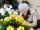Florist has 84 red and 48 white roses. How many same bouquets can he make from them if he must use all roses?
• Shepherd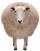The shepherd has fewer than 500 sheep; where they can be up to 2, 3, 4, 5, 6 row is always 1 remain, and as can be increased up to 7 rows of the sheep, and it is not increased no ovine. How many sheep has a shepherd?
• Janka and Danka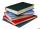Janka and Danka began to read the same day the books. Janka had 276 pages, Danka 204 pages. They agreed that both read every day the same number of pages until theirs books read. A) How many maximum pages they had to read the day to honor their agreement?

Do you have an exciting math question or word problem that you can't solve? Ask a question or post a math problem, and we can try to solve it.

We will send a solution to your e-mail address. Solved examples are also published here. Please enter the e-mail correctly and check whether you don't have a full mailbox.

Do you want to calculate greatest common divisor two or more numbers? Greatest common divisor (GCD) - math problems. Reason - math problems.